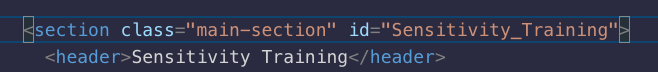# Each .main-section should have an id that matches the text of its first child, having any spaces in the child's text replaced with underscores (_) for the id’s

Tell us what’s happening:

``````<p>Example:</p>

<p>The following while loop iterates as long as n is less than three:</p>

<code id="label">var n = 0; var x = 0; while (n < 3) { n++; x += n; }</code>

<p>With each iteration, the loop increments n and adds that value to x. Therefore, x and n take on the following values:</p>

<ul>

<li>After the first pass: n = 1 and x = 1</li>

<li>After the second pass: n = 2 and x = 3</li>

<li>After the third pass: n = 3 and x = 6</li>

</ul>

<p>After completing the third pass, the condition n < 3 is no longer true, so the loop terminates.</p>

</section>

<section class="main-section" id="Function_declarations">

<p>A function definition (also called a function declaration, or function statement) consists of the function keyword, followed by:</p>

<ul>

<li>The name of the function.</li>

<li>A list of arguments to the function, enclosed in parentheses and separated by commas.</li>

<li>The JavaScript statements that define the function, enclosed in curly brackets, { }.</li>

</ul>

<p>For example, the following code defines a simple function named square:</p>

<code id="label">function square(number) { return number * number; }</code>

<p>The function square takes one argument, called number. The function consists of one statement that says to return the argument of the function (that is, number) multiplied by itself. The return statement specifies the value returned by the function.</p>

<code id="label">return number * number;</code>

<p>Primitive parameters (such as a number) are passed to functions by value; the value is passed to the function, but if the function changes the value of the parameter, this change is not reflected globally or in the calling function.</p>

</section>

<section class="main-section" id="Reference">

</section>

</main>

</body>

</html>
``````

WARNING

The challenge seed code and/or your solution exceeded the maximum length we can port over from the challenge.

You will need to take an additional step here so the code you wrote presents in an easy to read format.

Please copy/paste all the editor code showing in the challenge from where you just linked.

``````Replace these two sentences with your copied code.
Please leave the ``` line above and the ``` line below,
because they allow your code to properly format in the post.

``````

User Agent is: `Mozilla/5.0 (Macintosh; Intel Mac OS X 10_15_7) AppleWebKit/537.36 (KHTML, like Gecko) Chrome/101.0.4951.64 Safari/537.36`

Challenge: Build a Technical Documentation Page

are you sure, you/re not talking about two different things?!

your provided snippet and description is talking about some function but topic titles addresses some “html-css” issue!!

1 Like

which step?!

and try to be bit more “exact” when you’re asking for help, it might be super obvious to you, but for others it might not!! so help us to understand better what seems to be an issue here and where exactly, it gets easier that wayThe .main-section id needs to match the first child, which in this case is the header element. If there’s a space/ uppercase character in the header text, ensure that it’s identical to the .main-section id. I hope that helps!

1 Like

You can also use the “preformatted text” tool in the editor (`</>`) to add backticks around text.